# Running and Timing Programs¶

In this notebook, we'll learn how to write programs that can launch other programs and time how long it takes to do things (you'll often be combining these skills to time how long it takes to run a program).

Both these skills are covered in much more detail in an optional reading, Chapter 17 of Automate the Boring Stuff: https://automatetheboringstuff.com/2e/chapter17/. If you decide to read that, we recommend skipping the middle sections, "Multithreading" through "Project: Multithreaded XKCD Downloader"

## Running Programs¶

### Example 1: Running pwd¶

Remember that running the pwd program in a shell tells you what directly you're currently in. Let's write some Python code to run the pwd program automatically and capture the output. We'll do this with the check_output function in the subprocess module (https://docs.python.org/2/library/subprocess.html#subprocess.check_output) -- let's import that.

In :
from subprocess import check_output


In the simplest form, we can run pass a program name (as a string) to the function, which will capture and return the output:

In :
output = check_output("pwd")
output

Out:
b'/home/trh/lec3\n'

What type is that output? It looks like a string, but with a "b" in front. Hmmmm....

In :
type(output)

Out:
bytes

The bytes type in Python is a sequence, like a string. The difference is that bytes may contain letters (as in this case), or other types. If we know the encoding of a bytes sequence, we can convert to a string as follows:

In :
str_output = str(output, encoding="utf-8")
str_output

Out:
'/home/trh/lec3\n'
In :
type(str_output)

Out:
str

### Example 2: Checking Versions¶

What version of git do we have on this computer? From the command line, we could run git --version to find out. But let's do that in code. This is a little trickier because we have both a program name, git, and an argument, --version. The checkout_output function supports two ways of running programs with arguments.

Way 1: pass shell=True

In :
check_output("git --version", shell=True)

Out:
b'git version 2.17.1\n'

Or (preferred), we can pass the program and arguments in one list:

In :
check_output(["git", "--version"])

Out:
b'git version 2.17.1\n'

Let's actually do the string manipulation work to isolate the version:

In :
output = str(check_output(["git", "--version"]), encoding="utf-8")
output

Out:
'git version 2.17.1\n'
In :
parts = output.strip().split()
parts

Out:
['git', 'version', '2.17.1']
In :
version = parts[-1]
version

Out:
'2.17.1'

If we needed to have a specific version, we might use the above to have an assert like this:

assert version == 2.17.1

What if the program isn't installed, or we pass it some arguments that cause it to crash, as in the following example? We'll want to have catch some exceptions in these scenarios:

In :
import subprocess

try:
output = str(check_output(["git", "--oops"]), encoding="utf-8")
except FileNotFoundError:
print("program not installed?")
except subprocess.CalledProcessError as e:
print("program crashed")
# if there were any output before it crashed, we could look at it
# with this:
print("OUTPUT:", e.output)

program crashed
OUTPUT: b''


### Example 3: Making Animations¶

A common situation is that there will be some program that does something useful that we can't directly do in Python, and we'll want to write Python code to run these external programs to make use of their features.

For example, the ffmpeg program can make an animated video by glueing together a bunch of .png image files in sequence. There are ways to make animations directly in Python, but for now let's see how we can execute ffmpeg with check_output to make a video.

First, you should install the ffmpeg program on Ubuntu so we can use it -- run the following in the shell:

sudo apt install ffmpeg

Now, let's write some code to make a series of plots with a red dot in different positions, and save those plots as 0.png, 1.png, etc. The idea is that these images are similar enough that if you flipped through them, it would look like a rough video.

In :
import os
import matplotlib
from matplotlib import pyplot as plt

In :
%matplotlib inline

In :
matplotlib.rcParams["font.size"] = 16

In :
def plot_circle(filename, x, y):
fig, ax = plt.subplots()
ax.set_xlim(0, 1)
ax.set_ylim(0, 1)
ax.plot(x, y, 'ro', markersize=20)
fig.savefig(os.path.join("img", filename))

if not os.path.exists("img"):
os.mkdir("img")

plot_circle("0.png", x=0, y=0)
plot_circle("1.png", x=0.25, y=0.25)
plot_circle("2.png", x=0.5, y=0.5)
plot_circle("3.png", x=0.75, y=0.25)
plot_circle("4.png", x=1, y=0)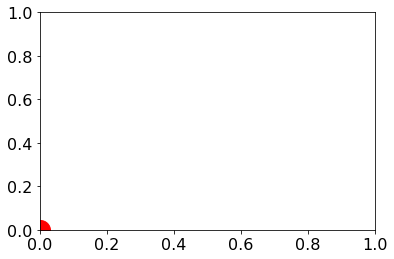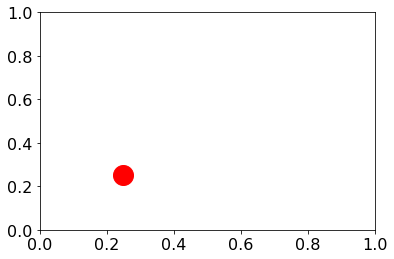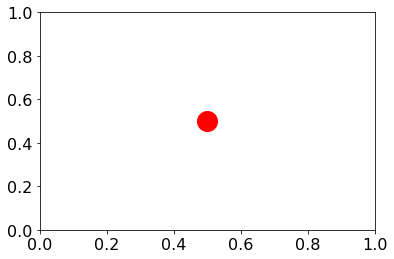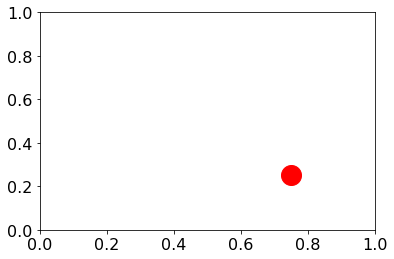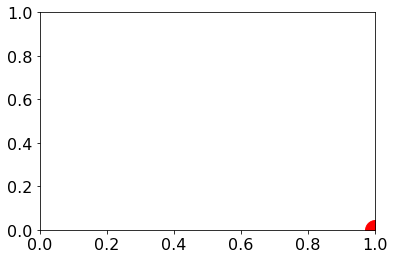Let's check that we created the png files in the img directory:

In :
os.listdir("img")

Out:
['0.png', '1.png', '4.png', '2.png', '3.png']

Let's also check that they look right. In the IPython.display module, there are Image(...) and HTML(...) functions that are useful for loading pictures and HTML directly into our notebook. Let's use the first function to check that our 0.png file looks right.

In :
from IPython.display import Image, HTML
Image(filename='img/0.png')

Out: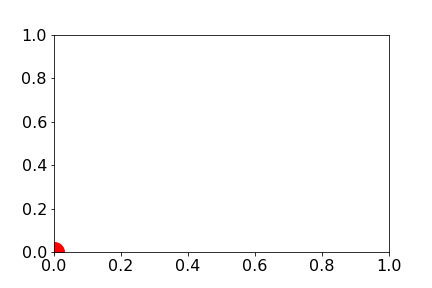Great! Now, from the command line, try running this command, inside the same directory where this notebook is running:

'ffmpeg -y -framerate 5 -i img/%d.png out.mp4

If it succeeds, there should be an out.mp4 file generated. Try downloading it to your computer via the Jupyter interface (don't try to open it directly from Jupyter) and open it on your laptop. Cool, huh?

Now let's try running that same command with check_output. We'll need to break up all the arguments into different entries in a list:

In :
check_output(['ffmpeg', '-y', '-framerate', '5', '-i', 'img/%d.png', 'out.mp4'])

Out:
b''

There was no output, which is fine. But it should have created an out.mp4 file, as before. You can embed .mp4 video files in websites with the <video> tag. This is great because we can inject HTML using the HTML(...) function from earlier:

In :
HTML("This is <b>bold</b> text.")

Out:
This is bold text.

The natural thing to do is to inject some HTML to embed the out.mp4 animation we just created:

In :
HTML("""
<video width="320" height="240" controls>
<source src="out.mp4" type="video/mp4">
</video>
""")

Out:

## Measuring Time¶

The easiest way to measure how long something takes is to check the time before and after we do it. We can check with the time function inside the time module. This function returns the number of seconds elapsed since Jan 1, 1970:

In :
import time
now = time.time()
now

Out:
1580137926.8447468
In :
minutes = now / 60
hours = minutes / 60
days = hours / 24
years = days / 365
years # should be about number of years since 1970 -- is it?

Out:
50.10584496590395

Let's use this to time how long a print call takes:

In :
before = time.time()
print("I'm printing something")
after = time.time()
print("It took", (after-before), "seconds to print")

I'm printing something
It took 0.0007390975952148438 seconds to print


A slightly cleaner version of the same that computers milliseconds (1ms is 1/1000 seconds):

In :
t0 = time.time()
print("I'm printing something")
t1 = time.time()
ms = (t1-t0) * 1000
print("It took", ms, "ms to print")

I'm printing something
It took 0.7138252258300781 ms to print


How long does it take to append something to the end of a list?

In :
L = []
t0 = time.time()
L.append("test")
t1 = time.time()
us = (t1-t0) * 1e6 # microseconds (there are 1 second has 1000000 microseconds)
print("microseconds:", us)

microseconds: 57.45887756347656


The problem with the above measurement is that it is it varies significantly each time you try it, and we can easily end up measuring something other than append time. For example, what if calling time.time() is much slower than calling L.append("test")? It is better to perform an operation many times between checking the start+stop times and then divide to get the average cost of the operation:

In :
L = []
append_count = 1000000 # do 1 million appends
t0 = time.time()
for i in range(append_count):
L.append("test")
t1 = time.time()
us = (t1-t0) / append_count * 1e6 # microseconds (there are 1 second has 1000000 microseconds)
print("microseconds:", us)

microseconds: 0.11862897872924805


### Example 1: the in operator¶

The in operator can be used to check whether a value is in a list or a set, but it's much faster on a set. If your code needs to perform the in operation a lot, this is a good reason to use a set rather than a list. Let's review how in works on each:

In :
L = ["A", "B", "C"]
S = {"A", "B", "C"}

In :
"A" in L, "D" in L, "A" in S, "D" in S

Out:
(True, False, True, False)

Let's see how fast in is if we are checking over 1 million numbers in a list or a set.

In :
seq_size = 1000000
L = list(range(seq_size))
S = set(range(seq_size))

# return average microseconds to perform lookup
def time_lookup(data, search):
trials = 1000
t0 = time.time()
for i in range(trials):
found = search in data
t1 = time.time()
return (t1-t0)*1e6/trials

time_lookup(L, 0), time_lookup(S, 0)

Out:
(0.03719329833984375, 0.03743171691894531)

Ok, looks like looking up 0 (the first number) is about equally fast in either data structure.

What if we lookup a number that's not stored?

In :
time_lookup(L, -1), time_lookup(S, -1)

Out:
(10068.64070892334, 0.0438690185546875)

Woah, now the list is >10K times slower! What if we lookup the last item in the list?

In :
time_lookup(L, 999999), time_lookup(S, 999999)

Out:
(11480.518341064453, 0.0591278076171875)

The set is fast again, but the list is still really slow (about as slow as looking up something that doesn't exist). What if we lookup a number in the middle?

In :
time_lookup(L, 500000), time_lookup(S, 500000)

Out:
(5739.615678787231, 0.05888938903808594)

Well, checking for something in the middle of a list is about twice as fast as checking for the last item. Can you guess why?

It turns out that while sets are designed around making in fast, running in on a list amounts to looping over ever item, much like a call to the following function.

In :
def is_in(L, search):
for item in L:
if search == L: # if this is True early in the list, the search is fast
return True
return False


How does the list size factor in when we perform an in and we don't find anything? Let's do an experiment to find out.

In :
from pandas import Series

times = Series()

for size in [1000, 2000, 5000, 10000]:
L = list(range(size))
microseconds = time_lookup(L, -1)
times.loc[size] = microseconds
times

Out:
1000      9.510994
2000     18.847942
5000     47.323465
10000    95.795155
dtype: float64
In :
ax = times.plot.line(color="r")

# following makes plot look better (only necessary if we plan to share it with others)
ax.spines["right"].set_visible(False)
ax.spines["top"].set_visible(False)
ax.set_xlabel("List Size")
ax.set_ylabel("Lookup Miss Time (μs)")
None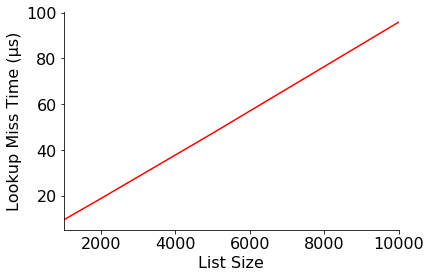Looking at the above, we would say that the in operator scales linearly. In otherwords, doubling the list size doubles the time it takes to perform the operation.

Not all functions we'll encounter will scale linearly. For example, consider this one, which checks whether the ratio of any two numbers in a list matches the ration we're searching for:

In :
def ratio_search(L, ratio):
for numerator in L:
for denominator in L:
if numerator / denominator == ratio:
return True
return False

ratio_search([1, 2, 3, 4], 0.75)

Out:
True
In :
ratio_search([1, 2, 3, 4], 0.2)

Out:
False

Let's see how it scales when we search for a ratio we know we won't find.

In :
import random, string

times = Series()

for i in range(6):
size = i * 1000
L = list(range(1, size+1)) # don't include 0, because we need to divide

t0 = time.time()
found = ratio_search(L, -1)
t1 = time.time()

times.loc[size] = t1-t0
times

Out:
0       0.000003
1000    0.056291
2000    0.222333
3000    0.499056
4000    0.890178
5000    1.397182
dtype: float64
In :
ax = times.plot.line(color="r")

# following makes plot look better (only necessary if we plan to share it with others)
ax.spines["right"].set_visible(False)
ax.spines["top"].set_visible(False)
ax.set_xlabel("List Size")
ax.set_ylabel("Lookup Miss Time (μs)")
None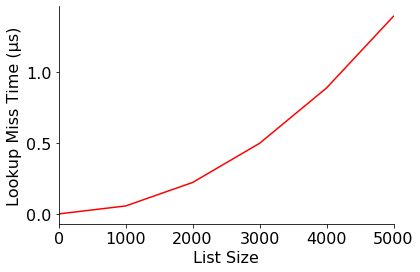The above is an example of quadratic scaling: doubling the list size quadruples the time it takes to run!

# Conclusion¶

In this notebook, we've learned how to automatically run programs and time code. Together, these skills provide the empirical basis for exploring performance and scalability. Soon, we'll be learning a bit of theory (complexity analysis) and notation (big-O) for thinking about what happens to performance as we add more data.

In [ ]: